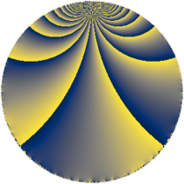# Properties

 Label 441.4.uLevel $441$ Weight $4$ Character orbit 441.u Rep. character $\chi_{441}(64,\cdot)$ Character field $\Q(\zeta_{7})$ Dimension $414$ Sturm bound $224$

# Related objects

## Defining parameters

 Level: $$N$$ $$=$$ $$441 = 3^{2} \cdot 7^{2}$$ Weight: $$k$$ $$=$$ $$4$$ Character orbit: $$[\chi]$$ $$=$$ 441.u (of order $$7$$ and degree $$6$$) Character conductor: $$\operatorname{cond}(\chi)$$ $$=$$ $$49$$ Character field: $$\Q(\zeta_{7})$$ Sturm bound: $$224$$

## Dimensions

The following table gives the dimensions of various subspaces of $$M_{4}(441, [\chi])$$.

Total New Old
Modular forms 1032 426 606
Cusp forms 984 414 570
Eisenstein series 48 12 36

## Trace form

 $$414q + 5q^{2} - 277q^{4} + 7q^{5} - 20q^{7} - 53q^{8} + O(q^{10})$$ $$414q + 5q^{2} - 277q^{4} + 7q^{5} - 20q^{7} - 53q^{8} - 39q^{10} - 93q^{11} + 21q^{13} - 231q^{14} - 1101q^{16} - 43q^{17} + 516q^{19} + 515q^{20} + 739q^{22} - 261q^{23} - 1266q^{25} - 381q^{26} + 62q^{28} + 243q^{29} + 516q^{31} + 141q^{32} - 1365q^{34} - 1071q^{35} + 345q^{37} + 665q^{38} + 1961q^{40} - 939q^{41} - 33q^{43} + 379q^{44} + 2433q^{46} + 1023q^{47} - 1408q^{49} + 6088q^{50} - 2715q^{52} - 331q^{53} + 853q^{55} + 854q^{56} - 3q^{58} - 1177q^{59} + 4399q^{61} - 3649q^{62} - 1491q^{64} + 707q^{65} + 912q^{67} - 5584q^{68} + 6559q^{70} + 3127q^{71} - 521q^{73} - 4505q^{74} + 2014q^{76} + 427q^{77} + 436q^{79} - 12464q^{80} + 1212q^{82} - 1131q^{83} + 161q^{85} + 633q^{86} + 827q^{88} + 7957q^{89} - 1113q^{91} + 6574q^{92} + 224q^{94} + 5222q^{95} + 268q^{97} + 10171q^{98} + O(q^{100})$$

## Decomposition of $$S_{4}^{\mathrm{new}}(441, [\chi])$$ into newform subspaces

The newforms in this space have not yet been added to the LMFDB.

## Decomposition of $$S_{4}^{\mathrm{old}}(441, [\chi])$$ into lower level spaces

$$S_{4}^{\mathrm{old}}(441, [\chi]) \cong$$ $$S_{4}^{\mathrm{new}}(49, [\chi])$$$$^{\oplus 3}$$$$\oplus$$$$S_{4}^{\mathrm{new}}(147, [\chi])$$$$^{\oplus 2}$$